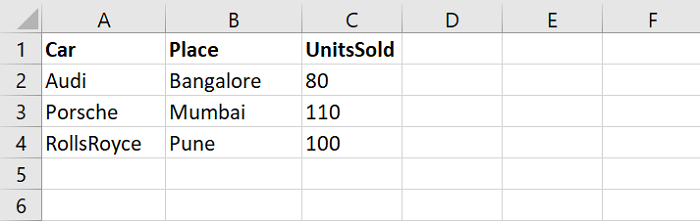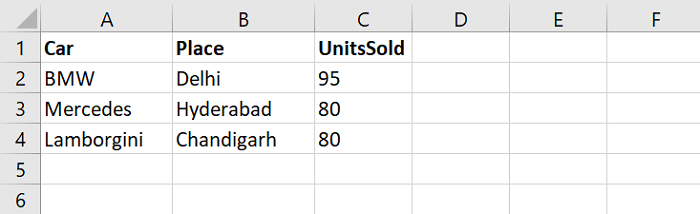# How to Merge multiple CSV Files into a single Pandas dataframe ?

PythonServer Side ProgrammingProgramming

To merge more than one CSV files into a single Pandas dataframe, use read_csv. At first, import the required Pandas library. Here. We have set pd as an alias −

import pandas as pd

Now, let’s say the following are our CSV Files −

Sales1.csvSales2.csvWe have set the path as string. Both the files are on the Desktop −

file1 = "C:\\Users\\amit_\\Desktop\\sales1.csv"
file2 = "C:\\Users\\amit_\\Desktop\\sales2.csv"

Next, merge the above two CSV files. The pd.concat() merge the CSV files together −

dataFrame = pd.concat(
map(pd.read_csv, [file1, file2]), ignore_index=True)

## Example

Following is the code −

import pandas as pd

file1 = "C:\\Users\\amit_\\Desktop\\sales1.csv"
file2 = "C:\\Users\\amit_\\Desktop\\sales2.csv"

print("Merging multiple CSV files...")

# merge
dataFrame = pd.concat(
print(dataFrame)

## Output

This will produce the following output −

          Car       Place   UnitsSold
0        Audi   Bangalore          80
1     Porsche      Mumbai         110
2  RollsRoyce        Pune         100
3         BMW       Delhi          95
5  Lamborgini  Chandigarh          80